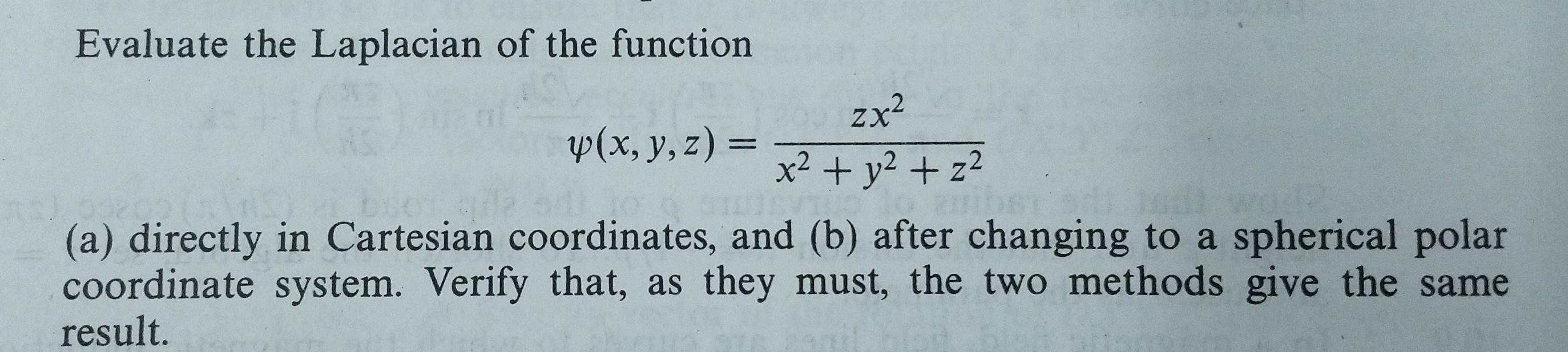Evaluate the Laplacian of the functionzx2x2 + y2+ z2p(x, y,z)-(a) directly in Cartesian coordinates, and (b) after changing to a spherical polarcoordinate system. Verify that, as they must, the two methods give the sameresult.

Questionhelp_outlineImage TranscriptioncloseEvaluate the Laplacian of the function zx2 x2 + y2+ z2 p(x, y,z)- (a) directly in Cartesian coordinates, and (b) after changing to a spherical polar coordinate system. Verify that, as they must, the two methods give the same result. fullscreen
Step 1

(a) Let us calculate the Laplacian of the function directly in Cartesian co-ordinates :

Step 2

Now, putting the values of Equation  (ll), (lll) and (IV) in Equation (l) and on simplifying, we get :

Step 3

(b) Let us change the function to a sph...

Want to see the full answer?

See Solution

Want to see this answer and more?

Our solutions are written by experts, many with advanced degrees, and available 24/7

See Solution
Tagged in

Math